# Create a recursive method that takes as input the root Node of a tree and returns true if the N v...

Create a recursive method that takes as input the root Node of a tree and returns true if the N variable of each node within that tree has the correct value (i.e., all nodes are labeled with the proper size of the subtree they define), and false otherwise.

Program :

import java.util.Scanner;
class BTNode{
BTNode left, right;
int data;
public BTNode(){
left = null;
right = null;
data = 0;
}
public BTNode(int n){
left = null;
right = null;
data = n;
}
public void setLeft(BTNode n){
left = n;
}
public void setRight(BTNode n){
right = n;
}
public BTNode getLeft(){
return left;
}
public BTNode getRight(){
return right;
}
public void setData(int d){
data = d;
}
public int getData()

{

return data;

}

}
class BT{
private BTNode root;
public BT(){
root = null;
}
public boolean isEmpty(){
return root == null;
}
public void insert(int data){
root = insert(root, data);
}
private BTNode insert(BTNode node, int data){
if (node == null)
node = new BTNode(data);
else{
if (node.getRight() == null)
node.right = insert(node.right, data);
else
node.left = insert(node.left, data);
}
return node;
}
public void inorder(){
inorder(root);
}
private void inorder(BTNode r){
if (r != null){
inorder(r.getLeft());
System.out.print(r.getData() +" ");
inorder(r.getRight());
}
}
public boolean search(int val){
return search(root, val);
}
private boolean search(BTNode r, int val){
if (r.getData() == val)
return true;
if (r.getLeft() != null)
if (search(r.getLeft(), val))
return true;
if (r.getRight() != null)
if (search(r.getRight(), val))
return true;
return false;

}
}

public class BinaryTree{
public static void main(String[] args){
Scanner scan = new Scanner(System.in);
BT bt = new BT();
char ch;
System.out.println("#############Binary Tree ###################");
do{
System.out.println("Enter integer element to insert :");
bt.insert(scan.nextInt());
System.out.print(" In order : ");
bt.inorder();
System.out.print(" ");
System.out.println(" Do you want to continue (Type y or n) : ");
ch = scan.next().charAt(0);
} while (ch == 'Y'|| ch == 'y');
System.out.println("Enter a node value to check is correct or not in the tree :");
int a = scan.nextInt();
System.out.println(bt.search(a));
}
}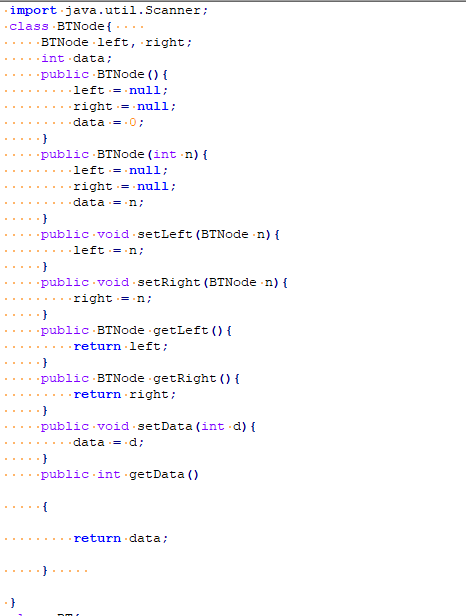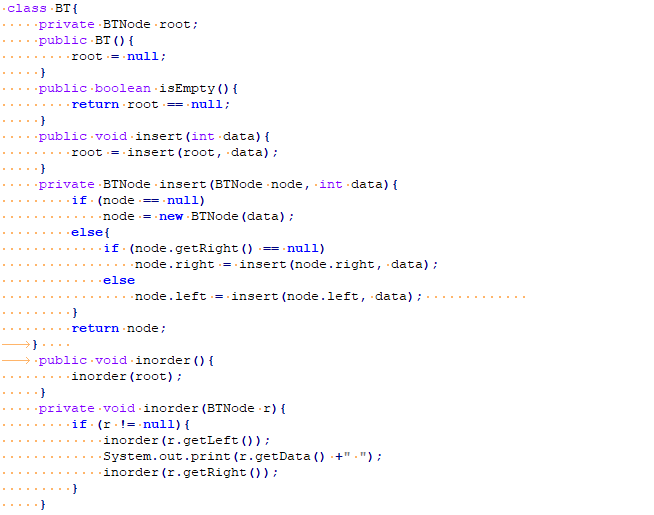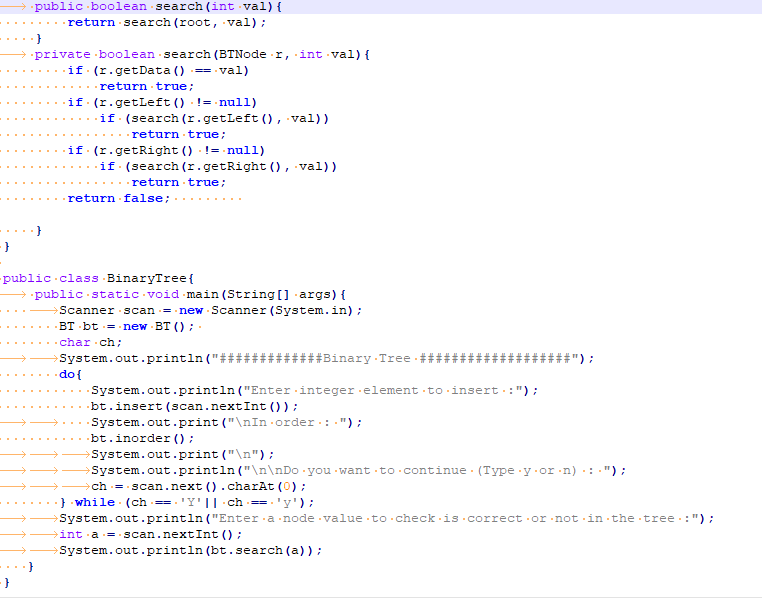Output :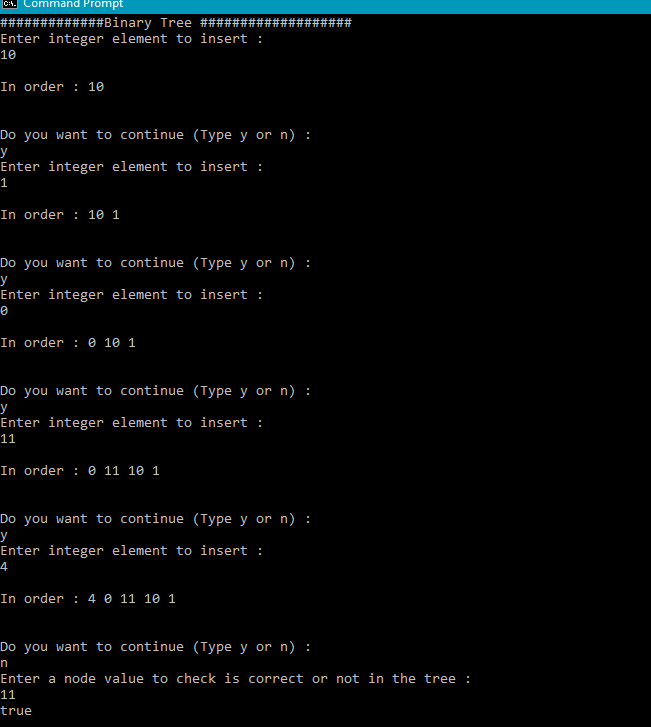##### Add Answer of: Create a recursive method that takes as input the root Node of a tree and returns true if the N v...
Similar Homework Help Questions
• ### 1. Write a recursive function that returns the sum of all even integers in a LinkedBinaryTree. Yo...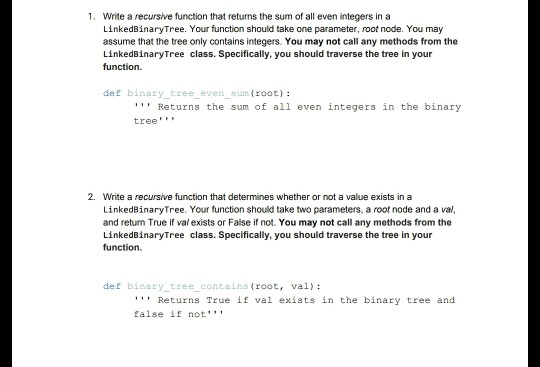please explain each line of code! ( in python ) 1. Write a recursive function that returns the sum of all even integers in a LinkedBinaryTree. Your function should take one parameter, root node. You may assume that the tree only contains integers. You may not call any methods from the LinkedBinaryTree class. Specifically, you should traverse the tree in your function def binary tree even sum (root): Returns the sum of al1 even integers in the binary tree 2....

• ### In this assignment, you will add several methods to the Binary Search Tree. You should have compl...

In this assignment, you will add several methods to the Binary Search Tree. You should have completed the following three methods in the lab: public void insert(Key key, Value value) public Value get(Key key) public void inorder(Node root) For this assignment, you will implement the following: public void remove(Node root, Key key) public Key getMin(Node n) public Key getMax(Node n) public int height(Node n) The main method contains the statements to check whether your implementation works. You need to change...

• ### need help creating a binary search tree ☆ Creating a Binary Search Tree Given atst of integers, implement the foll...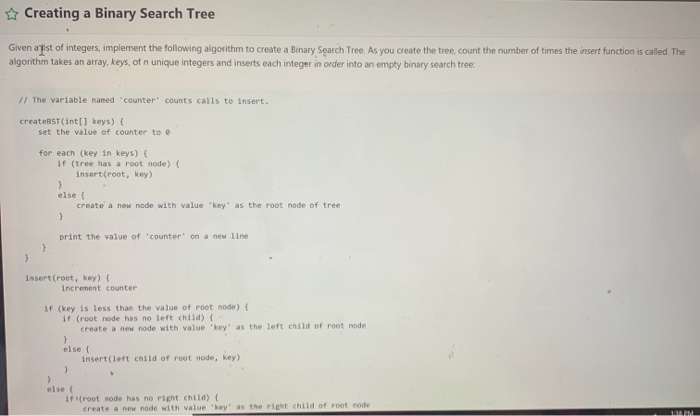need help creating a binary search tree ☆ Creating a Binary Search Tree Given atst of integers, implement the following algorithm to create a Binary Search Tree. As you create the tree, count the number of times the insert function is called Te algorithm takes an array, keys, of n unique integers and inserts each integer in order into an empty binary search tree / The variable named counter counts calls to insert. createBST (int[1 keys)( set the value of...

• ### generate an N-node random binary search tree with

x.øi5te a function to generate an N-node random binary search tree withdistinct keys L through N.What is the running time of the routine?I have this so far:template <typename Comparable>class BinarySearchTree{public:BinarySearchTree( );BinarySearchTree( const BinarySearchTree &rhs );//Destructor for the tree~BinarySearchTree( ){makeEmpty( );}const Comparable &findMin( ) const;const Comparable &findMax( ) const;//Returns true if x is found in the tree.bool contains( const Comparable &x ) const{return contains( x, root );}bool isEmpty( ) const;void printTree( ) const;void makeEmpty( );//Insert x into the tree; duplicates are...

• ### Write a binaryTree code method that returns true just if this binaryTree is equal to other...

Write a binaryTree method,bool equal(const BinaryTree &otherTree)const,that returns true just if this BinraryTree and otherThree are equal.Write a driver program that reads two binary trees from a file, writes the inorder and preorder traversals of the trees, and reports whether the two trees areequal.A header file containing definition and declaration of TreeNode and BinaryTree is as follows:// BinaryTree.h// A binary tree class that stores string in the nodes.// All code is implemented here in the .h file.#ifndef _BINARY_TREE_H_#define _BINARY_TREE_H_#include#include//*** TreeNode...

• ### Sc Python 1 Task 2 3 Consider a binary tree of N vertices 4 such that children of node K are 2* K + 1. Vertex 1...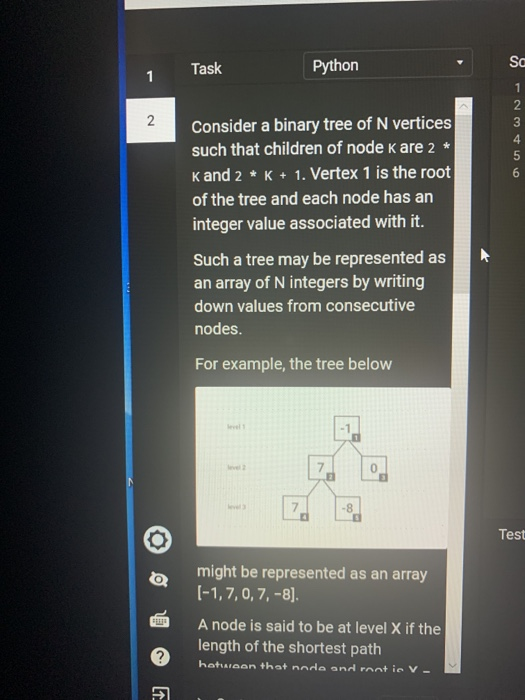Sc Python 1 Task 2 3 Consider a binary tree of N vertices 4 such that children of node K are 2* K + 1. Vertex 1 is the root Kand 2 of the tree and each node has an integer value associated with it. Such a tree may be represented as an array of N integers by writing down values from consecutive nodes For example, the tree below 8 Test might be represented as an array o A node...

• ### a. b. c. If the client requests the removal of a single node in a general...a. b. c. If the client requests the removal of a single node in a general tree (a tree with no limit on the number of children per node) as we have studied it, this might result in many more nodes than the one requested to be removed (from the client's perspective) While the answer will be the same for normal and lazy deletion, you can assume this tree does normal, not lazy, deletion, to avoid unnecessary thinking time. O...

• ### C++ Binary Search Tree question. I heed help with the level 2 question please, as level...C++ Binary Search Tree question. I heed help with the level 2 question please, as level 1 is already completed. I will rate the answer a 100% thumbs up. I really appreciate the help!. Thank you! searching.cpp #include <getopt.h> #include <iostream> #include <sstream> #include <stdlib.h> #include <unistd.h> using namespace std; // global variable for tree operations // use to control tree maintenance operations enum Mode { simple, randomised, avl } mode; // tree type // returns size of tree //...

• ### C++ Binary Search Tree question. I heed help with the level 2 question please, as level...C++ Binary Search Tree question. I heed help with the level 2 question please, as level 1 is already completed. I will rate the answer a 100% thumbs up. I really appreciate the help!. Thank you! searching.cpp #include <getopt.h> #include <iostream> #include <sstream> #include <stdlib.h> #include <unistd.h> using namespace std; // global variable for tree operations // use to control tree maintenance operations enum Mode { simple, randomised, avl } mode; // tree type // returns size of tree //...

• ### Does not pass the testcase testDTreeAdvancedReRootchangesParent Java I'm trying to extend the implementation of a general...Does not pass the testcase testDTreeAdvancedReRootchangesParent Java I'm trying to extend the implementation of a general tree with new operations that change the structure of the tree. I've created 5 classes: Node.java, SimpleNode.java, Tree.java, RerootableTree.java and SimpleTree.java(which is the main java class that I need to change). The code does not pass ONE TESTCASE : testDTreeAdvancedReRootchangesParent The code passes all the other testcases except theone mentioned above. This is because in the SimpleTree.java the method "reRoot(Node newRoot)" is most likely...

Need Online Homework Help?